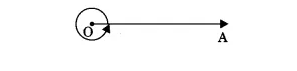Most Affordable JEE | NEET | 8,9,10 Preparation by Kota's Top IITian Doctor Faculties

# Lines and Angles Class 9 Maths Formulas

Class 9Hey, students are you looking for Lines and Angles Class 9 Maths Formulas? If yes. Then you are at the right place. In this post, I have listed all the formulas of Lines and Angles class 9 that you can use to learn and understand the concepts easily.

If you want to improve your class 9 Math, Lines and Angles concepts, then it is super important for you to learn and understand all the formulas.

By using these formulas you will learn about the Lines and Angles.

With the help of these formulas, you can revise the entire chapter easily.

## Lines and Angles Class 9 Maths Formulas

• Point: A point is a dot made by a sharp pen or pencil. It is represented by capital letter.
• Line: A straight and endless path on both the directions is called a line.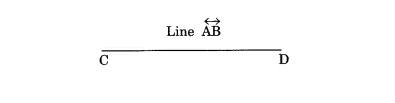• Line segment: A line segment is a straight path between two points.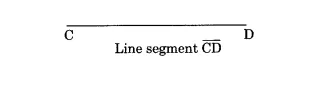• Ray: A ray is a straight path which goes forever in one direction.• Collinear points: If three or more than three points lie on the same line, then they are called collinear points.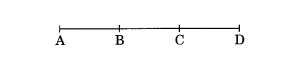• Non-collinear points: If three or more than three points does not lie on the same line, then they are called non-collinear points.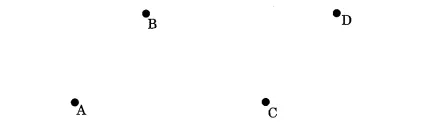• Angle: The space between two straight lines that diverge from a common point or between two planes that extend from a common line.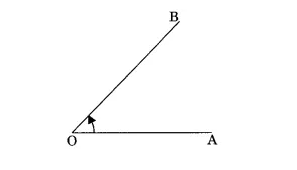Types of Angles

1. Acute angle: An angle between 0° and 90° is called acute angle.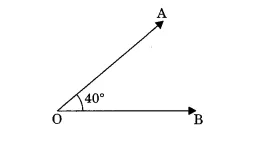2. Right angle: An angle which is equal to 90° is called right angle.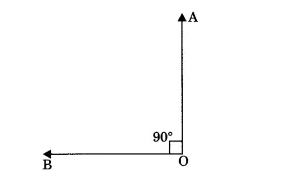3. Obtuse angle: An angle which is more than 90° but less than 180° is called obtuse angle.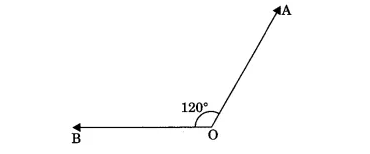4. Straight angle: An angle whose measure is 180° is called straight angle.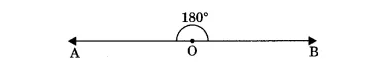5. Reflex angle: An angle whose measure is between 180° and 360° is called reflex angle.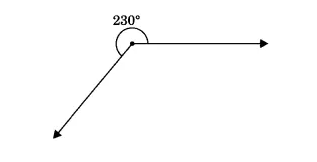6. Complete angle: An angle which is equal to 360° is called complete angle